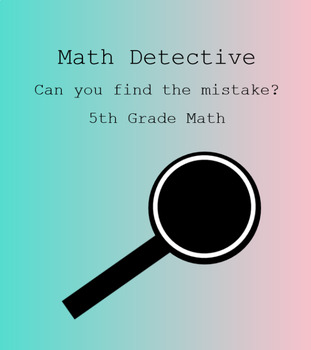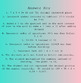Subject
Resource Type
File Type

NOTEBOOK (SMARTboard) File

(350 KB|12 pages)
Product Rating
Standards
• Product Description
• StandardsNEW

There are 10 slides covering place value, order of operations, multiplication and division including decimals that have common errors made by students. Students practice looking at the problem to see if they can determine the mistake that was made and if they can solve the problem correctly. It can be used altogether as one lesson or 1 problem a day.

Use parentheses, brackets, or braces in numerical expressions, and evaluate expressions with these symbols.
Add, subtract, multiply, and divide decimals to hundredths, using concrete models or drawings and strategies based on place value, properties of operations, and/or the relationship between addition and subtraction; relate the strategy to a written method and explain the reasoning used.
Find whole-number quotients of whole numbers with up to four-digit dividends and two-digit divisors, using strategies based on place value, the properties of operations, and/or the relationship between multiplication and division. Illustrate and explain the calculation by using equations, rectangular arrays, and/or area models.
Fluently multiply multi-digit whole numbers using the standard algorithm.
Read and write decimals to thousandths using base-ten numerals, number names, and expanded form, e.g., 347.392 = 3 × 100 + 4 × 10 + 7 × 1 + 3 × (1/10) + 9 × (1/100) + 2 × (1/1000).
Total Pages
12 pages
Included
Teaching Duration
N/A
Report this Resource to TpT
Reported resources will be reviewed by our team. Report this resource to let us know if this resource violates TpT’s content guidelines.